Subtle points about arithmetic

I agree with the result below.  If we multiply a number with 50 digits of
precision by a machine precision number, all the extra digits are garbage.  I
get back a number with 16 digits of precision and this is good.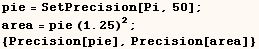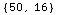Next I multiply a number with only 4 digits of precision by a machine
precision number and Mathematica doesn't know that the result should have 4
digits of precision.  I don't like this.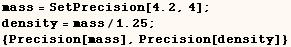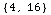To do the calculation above correctly we can do something like the line
below.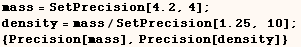At first you might guess that if a numeric function gets a machine precision
number it returns a machine precision number whenever possible.  This isn't
always true, and it can be hard to predict what you will get.  Consider the
line below, and look at the ByteCount of the results.  If memory is concern
and precision isn't important you should use N[Erf[x2]] to make sure the
result is a machine number.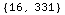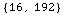In the line below (b1 = a1 + Pi - 1) and the kernel thinks (b1) has better
precision than (a1).  This is wrong!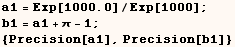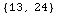WRI tech support  indicated the calculation above can be

done correctly by using (\$MinPrecision = -Infinity).  I can't recall how, but
I think I once saw that you can cause problems if you use the default setting
(\$MinPrecision=-∞).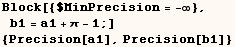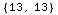Sometimes the kernel leaves machine precision arithmetic unfinished.  See the
example below.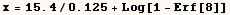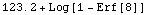Below we see simply using N on the result above returns Indeterminate.
That's why it was returned unfinished.  We have to use arbitrary precision to
get an ordinary number.  In this case I am impressed with how careful the
kernel is.Next we say x2=N[x,25], but we only get a machine number back because of the
123.2 term in (x).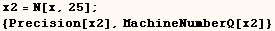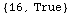Below I define x1 and x2 to be two machine numbers very close to 9.1.  Noticereturns True and (x2===9.1) returns False.  It's interesting that  (x1===9.1) returns True because we don't get zero for (x1-9.1).  Hence, if  you really want to know if two machine numbers are exactly the same you  should use (x-y===0.0) or (x-y==0.0) or (x-y==0).  I haven't  looked into the trade offs between the three forms.  It may be that neither  of these forms is sufficient if you are comparing arbitrary precision  numbers.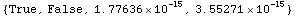In the cell above we saw that both x1 and x2 are a bit larger than 9.1.   In the next cell I define a value for f[9.1] and this definition is used for  f[x1] but not for f[x2].  It seems that when the kernel computes f[x] the  definition assigned to f[9.1] is used if and only if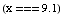evaluates to True.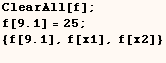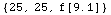For more read the  Numerics Report  in the Help Browser.

Created by Mathematica  (May 17, 2004)